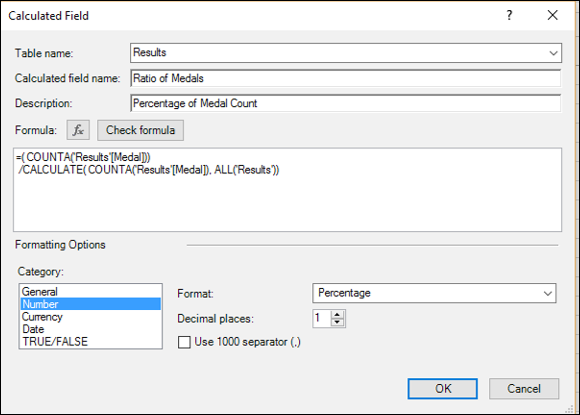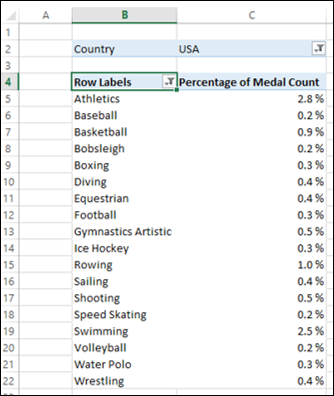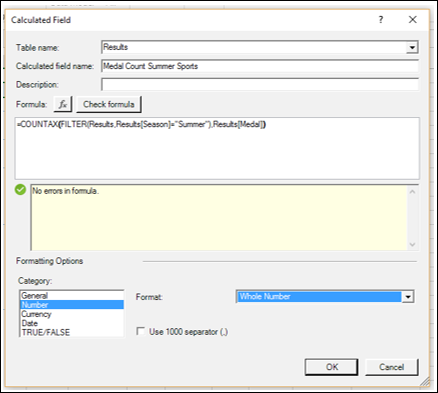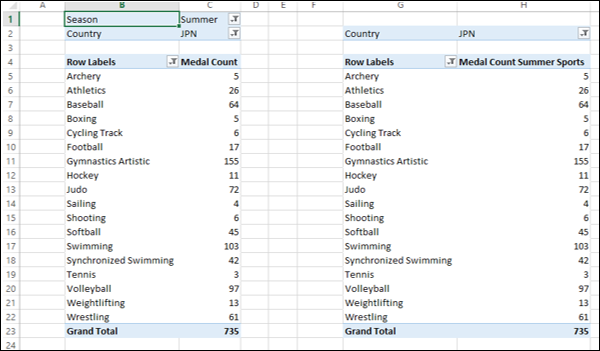# Excel DAX - Performing Complex Calculations

DAX formulas can perform complex calculations that involve custom aggregations, filtering, and the use of conditional values. You can do the following with DAX −

• Create custom calculations for a PivotTable.
• Apply a filter to a DAX formula.
• Remove filters selectively to create a dynamic ratio.
• Use a value from an outer loop.

## Creating Custom Calculations for a PivotTable

DAX functions CALCULATE and CALCULATETABLE are powerful and flexible. They are useful for defining calculated fields. These DAX functions enable you to change the context in which the calculation will be performed. You can also customize the type of aggregation or mathematical operation to perform.

### CALCULATE Function

CALCULATE (<expression>, [<filter1>], [<filter2>]…)

CALCULATE function evaluates the given expression in a context that is modified by zero or more specified filters.

If your data has been filtered, the CALCULATE function changes the context in which the data is filtered and evaluates the expression in the new context that you specify by the filters. That means, any existing filters on the specified column are removed and the filter used in the filter argument is applied instead.

### Example

Suppose you want to display the percentage of medals sport-wise filtered by Country names. Your calculation should get the percentage value overriding the filter that you apply on Country in the PivotTable.

• Define a calculated field – Percentage of Medal Count as shown in the following screenshot.With this DAX formula, all the rows in the Results table are taken into account in the CALCULATE function with the filter containing the ALL function. This way, you have the total count in the denominator.

Your PivotTable will be as shown in the following screenshot.In the above screenshot, Country is filtered to USA and Top 18 values are displayed in the PivotTable. Next, you can dynamically filter values in the PivotTable. However, the calculations will be correct by the custom DAX formula that you used.

The CALCULATETABLE function takes a table of values and performs the same action as that of CALCULATE function.

## Filtering Data in Formulas

You can create filters within DAX formulas, to select the values from the source data for use in calculations. You can do this by defining a filter expression and using it along with the table that is an input to the DAX formula.

The filter expression enables you to obtain a subset of the source data. The filter is applied dynamically each time that you update the results of the DAX formula, depending on the current context of your data and you can be assured of accurate and expected results.

The filter expression typically contains a DAX filter function that returns only selected rows of the table, which then can be used as an argument for another DAX function that you are using for data aggregation.

### Example

The following screenshot shows the definition of a calculated field that gives medal count only for summer sports.With this calculated field, the PivotTable looks as shown in the following screenshot.As you can observe, the values in the PivotTable on the right side with the new calculated field match with those that are in the PivotTable on the left side with the filter on Season field applied explicitly.

Note − DAX filter and value functions return a table, but never return the table or rows directly to the Data Model and hence are always embedded in another DAX function.

For details on these DAX functions, refer to the chapter – DAX Filter Functions.

### Adding and Removing Filters Dynamically

DAX Formulas that you use in a PivotTable can be affected by the PivotTable context. However, you can selectively change the context by adding or removing filters. You can use the DAX functions ALL and ALLEXCEPT to dynamically select the rows irrespective of the PivotTable context.

Additionally, you can use the DAX functions DISTINCT and VALUES for returning distinct values.

### Using a Value from an Outer Loop

You can use a value from a previous loop in creating a set of related calculations with DAX EARLIER function. This DAX function supports up to two levels of nested loops.